International
Tables for
Crystallography
Volume D
Physical properties of crystals
Edited by A. Authier

International Tables for Crystallography (2006). Vol. D, ch. 1.1, p. 7

## Section 1.1.3.1. Definition of a tensor

A. Authiera*

aInstitut de Minéralogie et de la Physique des Milieux Condensés, Bâtiment 7, 140 rue de Lourmel, 75015 Paris, France
Correspondence e-mail: aauthier@wanadoo.fr

#### 1.1.3.1. Definition of a tensor

| top | pdf |

For the mathematical definition of tensors, the reader may consult, for instance, Lichnerowicz (1947), Schwartz (1975)or Sands (1995).

#### 1.1.3.1.1. Linear forms

| top | pdf |

A linear form in the spaceis written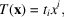where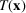is independent of the chosen basis and the's are the coordinates of T in the dual basis. Let us consider now a bilinear form in the product space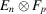of two vector spaces with n and p dimensions, respectively: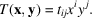The np quantities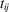's are, by definition, the components of a tensor of rank 2 and the form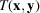is invariant if one changes the basis in the space. The tensoris said to be twice covariant. It is also possible to construct a bilinear form by replacing the spacesandby their respective conjugatesand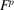. Thus, one writes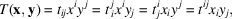where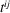is the doubly contravariant form of the tensor, whereas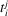andare mixed, once covariant and once contravariant.

We can generalize by defining in the same way tensors of rank 3 or higher by using trilinear or multilinear forms. A vector is a tensor of rank 1, and a scalar is a tensor of rank 0.

#### 1.1.3.1.2. Tensor product

| top | pdf |

Let us consider two vector spaces,with n dimensions andwith p dimensions, and let there be two linear forms,inand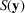in. We shall associate with these forms a bilinear form called a tensor product which belongs to the product space with np dimensions,: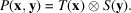This correspondence possesses the following properties:

 (i) it is distributive from the right and from the left; (ii) it is associative for multiplication by a scalar; (iii) the tensor products of the vectors with a basisand those with a basisconstitute a basis of the product space.

The analytical expression of the tensor product is then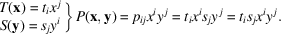One deduces from this that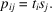It is a tensor of rank 2. One can equally well envisage the tensor product of more than two spaces, for example,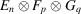in npq dimensions. We shall limit ourselves in this study to the case of affine tensors, which are defined in a space constructed from the product of the spacewith itself or with its conjugate. Thus, a tensor product of rank 3 will have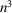components. The tensor product can be generalized as the product of multilinear forms. One can write, for example,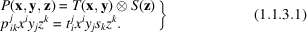### References

Lichnerowicz, A. (1947). Algèbre et analyse linéaires. Paris: Masson.
Sands, D. E. (1995). Vectors and tensors in crystallography. New York: Dover.
Schwartz, L. (1975). Les tenseurs. Paris: Hermann.